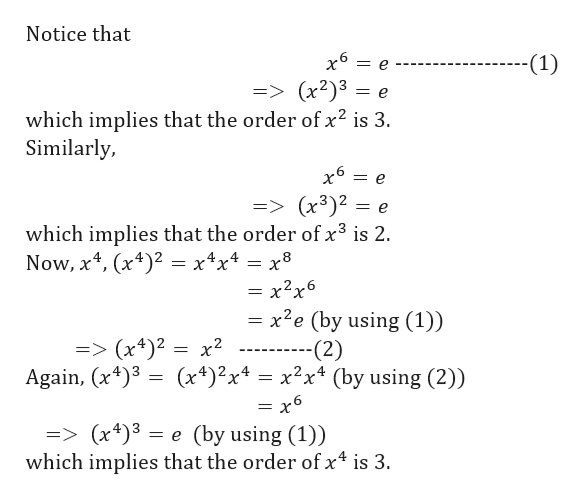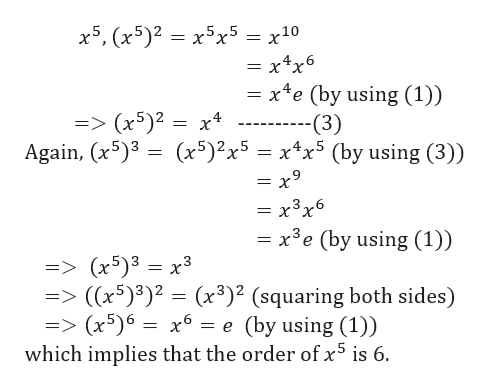Let x belong to a group and |x| = 6. Find |x^2|, |x^3|, |x^4|, and |x^5|. Let y belong to a group and |y|=9. Find |y^i| for i=2, 3, ...,8. Do these examples suggest any relationship between the order of the power of an element and the order of the element?

Question

Let x belong to a group and |x| = 6. Find |x^2|, |x^3|, |x^4|, and |x^5|. Let y belong to a group and |y|=9. Find |y^i| for i=2, 3, ...,8. Do these examples suggest any relationship between the order of the power of an element and the order of the element?

Step 1

Let G be the group, e be the identity element of G and x∈G such that |x|=6 or x6=e which means the order of x is 6.

First, we need to find the order of the elements x2, x3, x4 and x5.

Step 2

Now,help_outlineImage TranscriptioncloseNotice that х6 -(1) => (x2)3 which implies that the order of x2 is 3 Similarly, = e => (x3)2 which implies that the order of x3 is 2. Now, x4, (x4) = x4x4 = x8 = x2x6 = e = x2e (by using (1)) -(2) (x4)2x4x2x4 (by using (2)) => (x4)2 x2 Again, (x4)3 = x6 => (x4)3 which impliess that the order of x4 is 3 (by using (1)) = e fullscreen
Step 3

Again,

...help_outlineImage Transcriptionclosex5, (x5)2x5x5 = x10 = x4x6 = x e (by using (1)) x4 - =>(x5)2 = Again, (x53 -(3) (x5)2x5 x4x5 (by using (3)) = x9 = x3x6 = x3e (by using (1)) => (x5)3 x3 => ((x5)3)2 (x3)2 (squaring both sides) => (x5)6 which implies that the order of x5 is 6. = X (by using (1)) fullscreen

Want to see the full answer?

See Solution

Want to see this answer and more?

Our solutions are written by experts, many with advanced degrees, and available 24/7

See Solution
Tagged in

Math